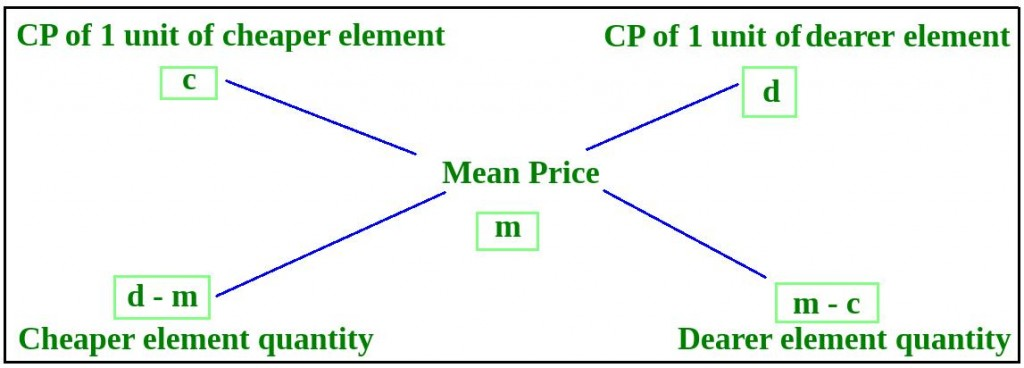# Program to solve the Alligation Problem

• Last Updated : 30 Aug, 2022

Write a program to find the ratio in which a shopkeeper will mix two types of rice worth Rs.kg and Rs.kg, so that the average cost of the mixture is Rs.kg.

Examples

Input: X = 50, Y = 70, Z = 65
Output: Ratio = 1:3

Input: X = 1000, Y = 2000, Z = 1400
Output: Ratio = 3:2

According to Alligation rule, the ratio of the weights of two items mixed will be inversely proportional to the deviation of attributes of these two items from the average attribute of the resultant mixture.

`w1 / w2 = (d - m) / (m - c)`Below program illustrate the above approach:

## C++

 `#include ``using` `namespace` `std;` `// Function to find the ratio of two mixtures``void` `alligation(``float` `x, ``float` `y, ``float` `m)``{``    ``// Find the cheaper among x and y``    ``float` `c = (x <= y) ? x : y;``    ``// Find the dearer among x and y``    ``float` `d = (x >= y) ? x : y;` `    ``// Find ratio r1:r2``    ``int` `r1 = d - m;``    ``int` `r2 = m - c;` `    ``// Convert the ration into simpler form``    ``int` `gcd = __gcd(r1, r2);` `    ``cout << r1 / gcd << ``":"` `<< r2 / gcd;``}` `// Driver code``int` `main()``{``    ``float` `x, y, z;``    ``x = 50;``    ``y = 70;``    ``z = 65;` `    ``alligation(x, y, z);` `    ``return` `0;``}`

## Java

 `// Java implementation of the``// above approach.``import` `java.util.*;` `class` `solution``{` `static` `float` `__gcd(``float` `a, ``float` `b)``{``    ``float` `dividend,divisor;``    ` `    ``// a is greater or equal to b``    ``if``(a>=b)``    ``dividend = a;``    ``else``    ``dividend = b;``    ` `    ``// b is greater or equal to a``    ``if``(a<=b)``    ``divisor = a;``    ``else``    ``divisor = b;``    ` `while``(divisor>``0``)``{``float` `remainder = dividend % divisor;``dividend = divisor;``divisor = remainder;` `}``return` `dividend;``}``// Function to find the ratio of two mixtures``static` `void` `alligation(``float` `x, ``float` `y, ``float` `m)``{``    ``// Find the cheaper among x and y``    ``float` `c;``    ``if` `(x <= y)``    ``c = x;``    ``else``    ``c = y;``    ``// Find the dearer among x and y``    ``float` `d ;``    ``if` `(x >= y)``    ``d = x;``    ``else``    ``d = y;` `    ``// Find ratio r1:r2``    ``float` `r1 = d - m;``    ``float` `r2 = m - c;` `    ``// Convert the ration into simpler form``    ``float` `gcd = __gcd(r1, r2);` `    ``System.out.println((``int``)(r1 / gcd)+``":"``+(``int``)(r2 / gcd));``}` `// Driver code``public` `static` `void` `main(String args[])``{``    ``float` `x, y, z;``    ``x = ``50``;``    ``y = ``70``;``    ``z = ``65``;` `    ``alligation(x, y, z);``}``}` `// This code is contributed by``// Shashank_sharma`

## Python3

 `# Python 3 implementation of the``# above approach.``from` `math ``import` `gcd` `# Function to find the ratio``# of two mixtures``def` `alligation(x, y, m):``    ` `    ``# Find the cheaper among x and y``    ``if` `(x <``=` `y):``        ``c ``=` `x``    ``else``:``        ``c ``=` `y``        ` `    ``# Find the dearer among x and y``    ``if` `(x >``=` `y):``        ``d ``=` `x``    ``else``:``        ``d ``=` `y` `    ``# Find ratio r1:r2``    ``r1 ``=` `d ``-` `m``    ``r2 ``=` `m ``-` `c` `    ``# Convert the ration into simpler form``    ``__gcd ``=` `gcd(r1, r2)` `    ``print``(r1 ``/``/` `__gcd, ``":"``, r2 ``/``/` `__gcd)` `# Driver code``if` `__name__ ``=``=` `'__main__'``:``    ``x ``=` `50``    ``y ``=` `70``    ``z ``=` `65` `    ``alligation(x, y, z)` `# This code is contributed by``# Surendra_Gangwar`

## C#

 `// C# implementation of the``// above approach.``using` `System;` `class` `GFG``{``    ``// Recursive function to return``    ``// gcd of a and b``    ``static` `int` `__gcd(``int` `a, ``int` `b)``    ``{``        ``// Everything divides 0``        ``if` `(a == 0)``            ``return` `b;``        ``if` `(b == 0)``            ``return` `a;``        ` `        ``// base case``        ``if` `(a == b)``            ``return` `a;``        ` `        ``// a is greater``        ``if` `(a > b)``            ``return` `__gcd(a - b, b);``        ``return` `__gcd(a, b - a);``    ``}``    ` `    ``// Function to find the ratio of``    ``// two mixtures``    ``static` `void` `alligation(``float` `x,``                           ``float` `y, ``float` `m)``    ``{``        ``// Find the cheaper among x and y``        ``float` `c = (x <= y) ? x : y;``        ` `        ``// Find the dearer among x and y``        ``float` `d = (x >= y) ? x : y;``    ` `        ``// Find ratio r1:r2``        ``int` `r1 = (``int``)(d - m);``        ``int` `r2 = (``int``)(m - c);``    ` `        ``// Convert the ration into``        ``// simpler form``        ``int` `gcd = __gcd(r1, r2);``    ` `        ``Console.Write(r1 / gcd + ``":"` `+``                      ``r2 / gcd);``    ``}``    ` `    ``// Driver code``    ``public` `static` `void` `Main()``    ``{``        ``float` `x, y, z;``        ``x = 50;``        ``y = 70;``        ``z = 65;``    ` `        ``alligation(x, y, z);``    ``}``}` `// This code is contributed``// by Akanksha Rai`

## PHP

 `= ``\$b``)``        ``\$dividend` `= ``\$a``;``    ``else``        ``\$dividend` `= ``\$b``;``    ` `    ``// b is greater or equal to a``    ``if``(``\$a` `<= ``\$b``)``        ``\$divisor` `= ``\$a``;``    ``else``        ``\$divisor` `= ``\$b``;``    ` `    ``while``(``\$divisor` `> 0)``    ``{``        ``\$remainder` `= ``\$dividend` `% ``\$divisor``;``        ``\$dividend` `= ``\$divisor``;``        ``\$divisor` `= ``\$remainder``;``    ``}``    ``return` `\$dividend``;``}` `// Function to find the ratio of``// two mixtures``function` `alligation(``\$x``, ``\$y``, ``\$m``)``{``    ``// Find the cheaper among x and y``    ``if` `(``\$x` `<= ``\$y``)``        ``\$c` `= ``\$x``;``    ``else``        ``\$c` `= ``\$y``;``    ` `    ``// Find the dearer among x and y``    ``if` `(``\$x` `>= ``\$y``)``        ``\$d` `= ``\$x``;``    ``else``        ``\$d` `= ``\$y``;` `    ``// Find ratio r1:r2``    ``\$r1` `= ``\$d` `- ``\$m``;``    ``\$r2` `= ``\$m` `- ``\$c``;` `    ``// Convert the ration into``    ``// simpler form``    ``\$gcd` `= __gcd(``\$r1``, ``\$r2``);` `    ``echo` `(int)(``\$r1` `/ ``\$gcd``) . ``":"` `.``         ``(int)(``\$r2` `/ ``\$gcd``);``}` `// Driver code``\$x` `= 50;``\$y` `= 70;``\$z` `= 65;` `alligation(``\$x``, ``\$y``, ``\$z``);` `// This code is contributed by``// Mukul Singh``?>`

## Javascript

 ``

Output:

`1:3`

Time Complexity: O(log(min(x,y)), gcd function takes logarithmic time complexity.
Auxiliary Space: O(1) as constant space is being used.

My Personal Notes arrow_drop_up